• 相关性分析可以发现不同变量之间相关性程度，本文以python为例进行实现。 1.相关性矩阵 corr = abs(data[['学科A','学科B','学科C','学科D','学业成败']].corr()) #abs取绝对值 corr 2.热力图可视化展示 from ...

相关性分析可以发现不同变量之间相关性程度，本文以python为例进行实现。

1.相关性矩阵
corr = abs(data[['学科A','学科B','学科C','学科D','学业成败']].corr()) #abs取绝对值
corr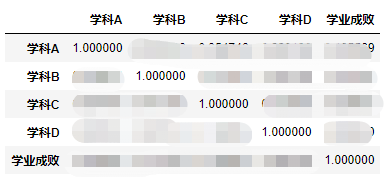2.热力图可视化展示
from matplotlib import pyplot as plt
import seaborn as sns
import numpy as np
fig = plt.figure()
names=['学科A','学科B','学科C','学科D','学业成败']
#fig.figsize:(40,40) #图片大小为20*20
# 以下代码用户显示中文
from pylab import *
mpl.rcParams['font.sans-serif'] = ['SimHei']
plt.rcParams['axes.unicode_minus']=False

plt.figure(figsize=(8,6))
ax = sns.heatmap(corr,cmap=plt.cm.Greys, linewidths=0.05,vmax=1, vmin=0 ,annot=True,annot_kws={'size':6,'weight':'bold'})
plt.xticks(np.arange(5)+0.5,names) #横坐标标注点
plt.yticks(np.arange(5)+0.5,names) #纵坐标标注点
plt.show()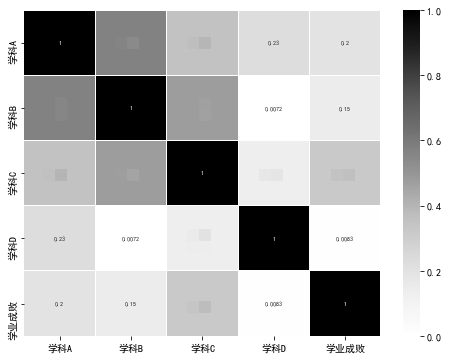展开全文可视化 数据可视化 机器学习
• ## Python相关性分析

千次阅读 2018-08-06 16:35:02
# -*- coding: utf-8 -*- """ Created on Thu Aug 2 11:17:06 2018 @author: 102155 """ def mean(x): return sum(x)/len(x) #计算每项数据与均值的差 ... return [x_i - x_bar f...
# -*- coding: utf-8 -*-
"""
Created on Thu Aug  2 11:17:06 2018

@author: 102155
"""

def mean(x):
return sum(x)/len(x)

#计算每项数据与均值的差
def de_mean(x):
x_bar = mean(x)
return [x_i - x_bar for x_i in x]

#辅助计算函数
#dot(v,w)点乘 v=[1,2,3] w=[7,8,9] dot(v,w) = 1*7 + 2*8 + 3*9]
def dot(v,w):
return sum(v_i*w_i for v_i,w_i in zip(v,w))
#v数组内的元素平方后相加
def sum_of_squares(v):
return dot(v,v)
#返回x数组的样本方差
def variance(x):
n = len(x)
deviations = de_mean(x)
return sum_of_squares(deviations)/(n-1)

#标准差
import math
def standard_deviation(x):
return math.sqrt(variance(x))

#协方差
def covariance(x,y):
n = len(x)
return dot(de_mean(x), de_mean(y))/(n-1)

#相关系数
def correlation(x,y):
std_x = standard_deviation(x)
std_y = standard_deviation(y)
if std_x>0 and std_y>0:
return covariance(x, y) / (std_x * std_y)
else:
return 0

import random

a = [random.randint(0, 100) for a in range(20)]
b = [random.randint(0, 100) for a in range(20)]

print(correlation(a,b))


展开全文• ## Python 数据相关性分析

万次阅读 多人点赞 2018-04-25 15:09:49
可能是此增彼涨，或者是负相关，也可能是没有关联，那么我们就需要一种能把这种关联性定量的工具来对数据进行分析，从而给我们的决策提供支持，本文即介绍如何使用 Python 进行数据相关性分析。 关键词 python 方差...

本文有视频教程，感兴趣的朋友可以前往观看 Python入坑实战系列 Part-2 - 简单数据相关性分析

概述
在我们的工作中，会有一个这样的场景，有若干数据罗列在我们的面前，这组数据相互之间可能会存在一些联系，可能是此增彼涨，或者是负相关，也可能是没有关联，那么我们就需要一种能把这种关联性定量的工具来对数据进行分析，从而给我们的决策提供支持，本文即介绍如何使用 Python 进行数据相关性分析。 关键词 python 方差 协方差 相关系数 离散度 pandas numpy
实验数据准备
接下来，我们将使用 Anaconda 的 ipython 来演示如何使用 Python 数据相关性分析，我所使用的 Python 版本为 3.6.2 。
首先，我们将会创建两个数组，数组内含有 20 个数据，均为 [0, 100] 区间内随机生成。
a = [random.randint(0, 100) for a in range(20)]
b = [random.randint(0, 100) for a in range(20)]
print(a)
>> [35, 2, 75, 72, 55, 77, 69, 83, 3, 46, 31, 91, 72, 12, 15, 20, 39, 18, 57, 49]
print(b)
>> [25, 24, 72, 91, 27, 44, 85, 21, 0, 64, 44, 31, 6, 91, 1, 61, 5, 39, 24, 43]

期望
在进行相关性分析之前，我们需要先为最终的计算分析做好准备。我们在分析前，第一个准备的是计算数据的期望。对于期望的定义，离散变量和连续变量是不一样的，具体定义如下：
对于连续随机变量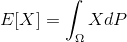在离散随机变量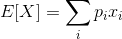在一般情况下，我们通过实验或者调查统计获取的数据很大一部分都属于离散随机变量，那么这里的期望我们也可以简单的理解为平均数，那么既然是平均数，那么我们就可以非常简单编写一个计算离散变量的期望的函数了。
def mean(x):
return sum(x) / len(x)
mean(a)
>> 46.05
mean(b)
>> 39.9

离散度 - 方差与标准差
接下来，我们需要计算的是数据的离散程度，在统计上，我们通常会使用方差和标准差来描述。 方差和期望一样，对于连续和离散的随机变量有着不同的定义，具体定义如下：
对于连续随机变量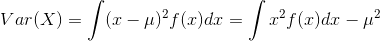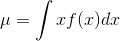对于离散随机变量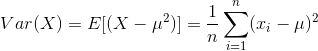与期望类似，这里我们一般只考虑离散变量的方差。还有一点值得注意，我们上面的离散变量方差公式，最后是除以 n ，但实际上，我们计算样本方差的时候一般会使用 n-1 ，具体原因可以参考知乎 《为什么样本方差（sample variance）的分母是 n-1？》。
而标准差，就是方差的平方根。那么，我们也可以像上面计算期望一样，给方差和标准差编写函数。
# 计算每一项数据与均值的差
def de_mean(x):
x_bar = mean(x)
return [x_i - x_bar for x_i in x]
# 辅助计算函数 dot product 、sum_of_squares
def dot(v, w):
return sum(v_i * w_i for v_i, w_i in zip(v, w))
def sum_of_squares(v):
return dot(v, v)
# 方差
def variance(x):
n = len(x)
deviations = de_mean(x)
return sum_of_squares(deviations) / (n - 1)
# 标准差
import math
def standard_deviation(x):
return math.sqrt(variance(x))

variance(a)
>> 791.8394736842105
varance(b)
>> 850.5157894736841

协方差与相关系数
接下来，我们进入正题，我们开始计算两组数据的相关性。我们一般采用相关系数来描述两组数据的相关性，而相关系数则是由协方差除以两个变量的标准差而得，相关系数的取值会在 [-1, 1] 之间，-1 表示完全负相关，1 表示完全相关。接下来，我们看一下协方差和相关系数的定义：
协方差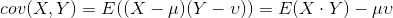相关系数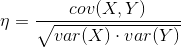同样的，我们根据上述的公式编写函数。
# 协方差
def covariance(x, y):
n = len(x)
return dot(de_mean(x), de_mean(y)) / (n -1)
# 相关系数
def correlation(x, y):
stdev_x = standard_deviation(x)
stdev_y = standard_deviation(y)
if stdev_x > 0 and stdev_y > 0:
return covariance(x, y) / stdev_x / stdev_y
else:
return 0

covariance(a, b)
>> 150.95263157894735
correlation(a, b)
>> 0.18394200852440826

根据上面的结果，相关系数为 0.18，可以推断这两组随机数有弱正相关。当然，我们知道，这两组数据都是使用 random 函数随机生成出来的，其实并没有什么相关性，这也是在数据处理中，需要特别留意的一个地方，统计的方法可以给我们一个定量的数值可供分析，但实际的分析也需要结合实际以及更多的情况综合考虑。
使用 numpy 计算协方差矩阵 相关系数
一般我们日常工作，都不会像上面一样把什么期望、方差、协方差一类的函数都重新写一遍，上面的代码只是让我们对这些计算更加熟悉。我们通常情况下会使用 numpy 一类封装好的函数，以下将演示一下如何使用 numpy 计算协方差。
import numpy as np
# 先构造一个矩阵
ab = np.array([a, b])
# 计算协方差矩阵
np.cov(ab)
>> array([[ 791.83947368,  150.95263158],
[ 150.95263158,  850.51578947]])

这里我们可以看到，这里使用 np.cov 函数，输出的结果是一个矩阵，这就是协方差矩阵。协方差矩阵数据的看法也不难，我们可以以上面的结果为例，矩阵1行1列，表示的是 a 数据的方差，这和我们上面的计算结果一致，然后1行2列和2行1列分别是 a b 以及 b a 的协方差，所以他们的值是一样的，然后最后2行2列就是 b 数据的方差。
接下来，我们继续使用 numpy 计算相关系数
np.corrcoef(ab)
>> array([[ 1.        ,  0.18394201],
[ 0.18394201,  1.        ]])

计算相关系数，我们使用 numpy 的 corrcoef 函数，这里的输出也是一个矩阵，这个矩阵数据的含义同上面的协方差类似，我们可以看到，这里我们的相关系数是 0.18 ，和我们上面自己编写的函数计算的结果一致。
使用 pandas 计算协方差、相关系数
除了使用 numpy，我们比较常用的 python 数据处理库还有 pandas，很多金融数据分析的框架都会使用 pandas 库，以下将演示如何使用 pandas 库计算协方差和相关系数。
import pandas as pd
# 使用 DataFrame 作为数据结构，为方便计算，我们会将 ab 矩阵转置
dfab = pd.DataFrame(ab.T, columns=['A', 'B'])
# A B 协方差
dfab.A.cov(dfab.B)
>> 150.95263157894738
# A B 相关系数
dfab.A.corr(dfab.B)
>>  0.18394200852440828
dfab
>>    A   B
0   35  25
1    2  24
2   75  72
3   72  91
4   55  27
5   77  44
6   69  85
7   83  21
8    3   0
9   46  64
10  31  44
11  91  31
12  72   6
13  12  91
14  15   1
15  20  61
16  39   5
17  18  39
18  57  24
19  49  43

可以看到，和 numpy 相比，pandas 对于有多组数据的协方差、相关系数的计算比 numpy 更为简便、清晰，我们可以指定计算具体的两组数据的协方差、相关系数，这样就不需要再分析结果的协方差矩阵了。
小结
本文通过创建两组随机的数组，然后通过参考定义公式编写函数，再到使用 numpy 以及 pandas 进行协方差、相关系数的计算。到这里我们应该已经了解了数据相关性分析的原理，以及简单的具体实践使用方法，日后在工作中遇到需要做数据相关性分析的时候，就可以派上用场了。
参考资料
《数据科学入门.格鲁斯 (Joel Grus).人民邮电出版社》
展开全文pandas numpy
• 常用相关性分析方法在分析特征间相关性时，常使用的方法是 pandas.DataFrame.corr ：DataFrame.corr(self, method=’pearson’, min_periods=1)其中包含的方法主要为：pearson：Pearson相关系数kendall：Kendall秩...

常用相关性分析方法
在分析特征间相关性时，常使用的方法是 pandas.DataFrame.corr ：
DataFrame.corr(self, method=’pearson’, min_periods=1)
其中包含的方法主要为：
pearson：Pearson相关系数kendall：Kendall秩相关系数Spearman：Spearman等级相关系数
Pearson相关系数
Pearson相关系数是一种最简单的，能帮助理解特征和响应变量之间关系的方法，该方法衡量的是变量之间的线性相关性，结果的取值区间为[-1，1]，-1表示完全的负相关(这个变量下降，那个就会上升)，+1表示完全的正相关，0表示没有线性相关。
Pearson Correlation速度快、易于计算，经常在拿到数据(经过清洗和特征提取之后的)之后第一时间就执行。 Scipy的pearsonr方法 能够同时计算相关系数和p-value。
import numpy as npfrom scipy.stats import pearsonr np.random.seed(0)size = 300x = np.random.normal(0, 1, size)print("Lower noise", pearsonr(x, x + np.random.normal(0, 1, size)))print("Higher noise", pearsonr(x, x + np.random.normal(0, 10, size))) # output:# Lower noise (0.7182483686213842, 7.324017312997672e-49)# Higher noise (0.05796429207933815, 0.31700993885325246)
这个例子中，我们比较了变量在加入噪音之前和之后的差异。当噪音比较小的时候，相关性很强，p-value很低。
Pearson相关系数的一个明显缺陷是，作为特征排序机制，他只对线性关系敏感。如果关系是非线性的，即便两个变量具有一一对应的关系，Pearson相关性也可能会接近0。
import numpy as npfrom scipy.stats import pearsonr x = np.random.uniform(-1, 1, 100000)print(pearsonr(x, x ** 2)) # output:# (0.0020775478403054566, 0.511199686284783)
Kendall秩相关系数
在统计学中，肯德尔相关系数是以 Maurice Kendall 命名的，并经常用希腊字母τ(tau)表示其值。肯德尔相关系数是一个用来测量两个随机变量相关性的统计值。一个肯德尔检验是一个无参数假设检验，它使用计算而得的相关系数去检验两个随机变量的统计依赖性。肯德尔相关系数的取值范围在-1到1之间，当τ为1时，表示两个随机变量拥有一致的等级相关性；当τ为-1时，表示两个随机变量拥有完全相反的等级相关性；当τ为0时，表示两个随机变量是相互独立的。
原始的 Kendall 秩相关系数定义在一致对 (concordant pairs) 和分歧对 (discordant pairs) 的概念上。所谓一致对，就是两个变量取值的相对关系一致；分歧对则是指它们的相对关系不一致。这么说有点难以理解，简单的说就是将两个变量进行排序，判断两者的排序值是否一致。如果一致则为1，如果倒叙则为-1。 Scipy的scipy.stats.kendalltau 提供的秩相关的检测方法：
from scipy.stats import kendalltau x1 = [12, 2, 1, 12, 2]x2 = [1, 4, 7, 1, 0]tau, p_value = kendalltau(x1, x2)print(tau, p_value) # output# -0.4714045207910316 0.2827454599327748
肯德尔相关系数与斯皮尔曼相关系数对数据条件的要求相同。针对非线性数据有一定的误差。
Spearman等级相关系数
在统计学中，斯皮尔曼等级相关系数以Charles Spearman命名，并经常用希腊字母ρ(rho)表示其值。斯皮尔曼等级相关系数用来估计两个变量X、Y之间的相关性，其中变量间的相关性可以使用单调函数来描述。如果两个变量取值的两个集合中均不存在相同的两个元素，那么，当其中一个变量可以表示为另一个变量的很好的单调函数时(即两个变量的变化趋势相同)，两个变量之间的ρ可以达到+1或-1。
斯皮尔曼等级相关系数对数据条件的要求没有皮尔逊相关系数严格，只要两个变量的观测值是成对的等级评定资料，或者是由连续变量观测资料转化得到的等级资料，不论两个变量的总体分布形态、样本容量的大小如何，都可以用斯皮尔曼等级相关系数来进行研究。对于服从Pearson相关系数的数据亦可计算Spearman相关系数，但统计效能要低一些。Pearson相关系数的计算公式可以完全套用 Spearman相关系数计算公式，但公式中的x和y用相应的秩次代替即可。 scipy.stats.spearmanr ：
from scipy.stats import spearmanr x1 = [1, 2, 3, 4, 5]x2 = [5, 6, 7, 8, 7]rho, p_value = spearmanr(x1, x2)print(rho, p_value) # output# 0.8207826816681233 0.08858700531354381
相关性检测 Python 实战：
import pandas as pdimport seaborn as snsimport matplotlib.pyplot as plt df = pd.read_excel("data/train.xlsx") plt.figure(figsize=(15, 13))sns.heatmap(df.corr(method="pearson"), annot=True, vmin=-1, vmax=1, cmap='coolwarm', fmt=".2f")plt.title("pearson")plt.savefig('corr_pearson.png') plt.figure(figsize=(15, 13))sns.heatmap(df.corr(method="kendall"), annot=True, vmin=-1, vmax=1, cmap='coolwarm', fmt=".2f")plt.title("kendall")plt.savefig('corr_kendall.png') plt.figure(figsize=(15, 13))sns.heatmap(df.corr(method="spearman"), annot=True, vmin=-1, vmax=1, cmap='coolwarm', fmt=".2f")plt.title("spearman")plt.savefig('corr_spearman.png')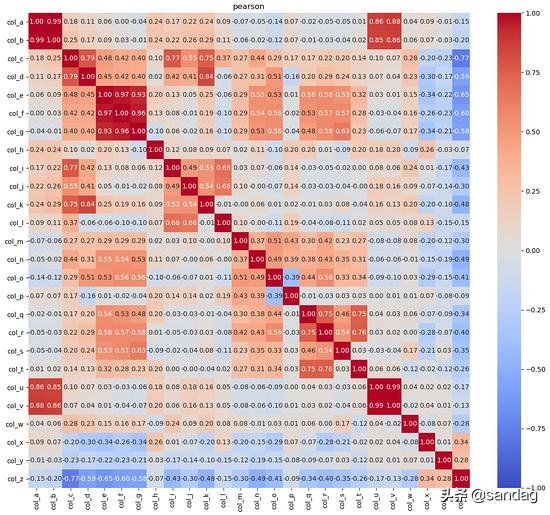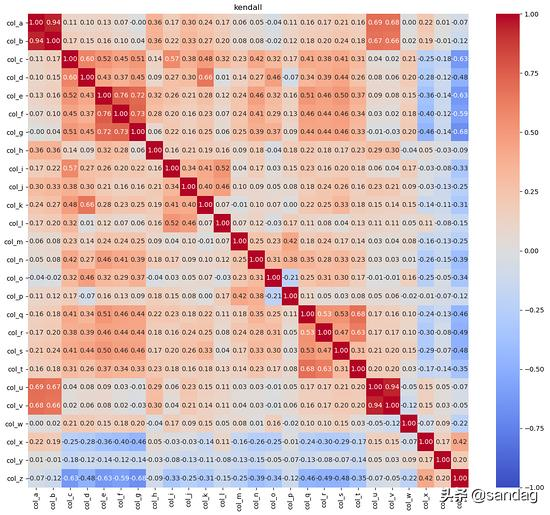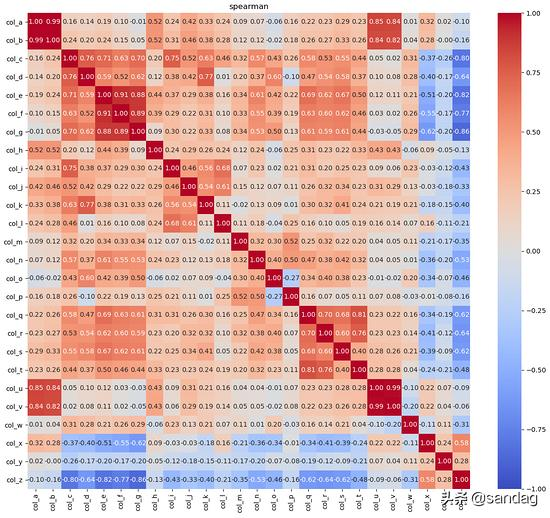相关性分析Predictive Power Score(PPS)
常用相关性分析存在的问题
1、有许多非线性的关系是分数根本无法检测到的，比如下图：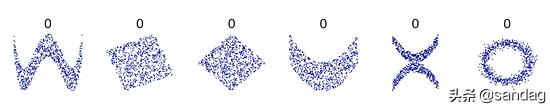2、计算出来的矩阵是对称的，即a与b的相关性同b与a的相同。更多的时候，关系是不对称的。一个有3个唯一值的列永远不可能完美地预测另一个有100个唯一值的列。 但事实可能恰恰相反。 显然，不对称很重要，因为它在现实世界中非常普遍。
Predictive Power Score (PPS)的实现逻辑
假设我们有两个列，想要计算 a 预测 b 的预测能力得分。 在这种情况下，我们将 b 视为目标变量，将 a 视为(唯一)特性。 我们现在可以计算一个交叉验证的决策树，并计算一个合适的评估度量。 当目标是数值时，我们可以使用决策树回归模型计算平均绝对误差(MAE)。 当目标是分类的，我们可以使用一个决策树分类器，并计算加权 F1。 你也可以使用其他的分数，比如 ROC 等。
PPS使用示例(Python)
import pandas as pdimport seaborn as snsimport matplotlib.pyplot as pltimport ppscore as pps df = pd.read_excel("data/train.xlsx") plt.figure(figsize=(15, 13))sns.heatmap(pps.matrix(df), annot=True, vmin=0, vmax=1, cmap='Blues', fmt=".2f")plt.title("Predictive Power Score")plt.savefig('corr_pps.png')
执行结果：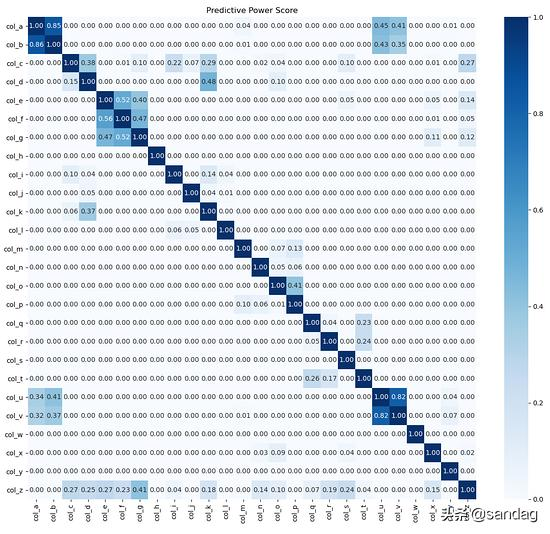展开全文• 接下来，我们将使用 Anaconda 的 ipython 来演示如何使用 Python 数据相关性分析，我所使用的 Python 版本为 3.6.2 。 首先，我们将会创建两个数组，数组内含有 20 个数据，均为 [0, 100] 区间内随机生成。 a = ...
• Python特征分析- 相关性分析相关性分析引入库图示初判变量之间的线性相关性散点图矩阵初判多变量间关系Pearson相关系数创建样本数据正态性检验 → pvalue >0.05制作Pearson相关系数求值表求出rPearson相关系数 - ...
• def dataPlot(): ''' 基于相关性系数计算结果来绘制 ''' data1 = [[1,3,1,1/5,1/2,3],[1/3,1,2,1/3,1,2],[1,1/2,1,1/5,1/2,5],[5,3,5,1,3,7],[2,1,2,1/3,1,3],[1/3,1/2,1/5,1/7,1/3,1]] data2 = [[0....
• ## 用 Python 对数据进行相关性分析

万次阅读 多人点赞 2020-07-08 08:32:00
在进行数据分析时，我们所用到的数据往往都不是一维的，而这些数据在分析时难度就增加了不少，因为我们需要考虑维度之间的关系。而这些维度关系的分析就需要用一些方法来进行衡量，相关性分析就是其中...
• 三、兼谈假设检 假设检验的3种类型 假设检验的套路： 四、利用Python进行相关性分析 判定两者相关的方式有两种： 图形观测法：通过绘制散点图判断两者是否存在一定相关关系 科学计算法：通过计算相关性系数r 我们用...
• 相关性分析是指对两个或多个具备相关性的变量元素进行分析，从而衡量两个变量因素的相关密切程度 一般常用四种方法： - 画图判断 - pearson（皮尔逊）相关系数 - sperman（斯皮尔曼）相关系数 - Cosine similarity ...
• 分析特征之间的相关性，得到哪个两个特征的具有关系，这样对于后期的数据分析可以提供帮助。 数据源示例： （每列都是一个特征，每行为一个用户） [10, 0, 0, 0, 0, 0, 0, 0, 0, 0, 0, 0, 0, 1, 0, 0, 0, 0, 5, 0]...
• 一、分析背景根据小红书的部分用户数据以及消费行为数据，使用Python建立线性回归模型，找到对用户消费影响较大的因素，预测用户的消费金额变化。根据模型，确定销售额较高用户的相关特征，并由此提出营销方案建议。...
• 主要介绍了Python中的相关分析correlation analysis的实现，文中通过示例代码介绍的非常详细，对大家的学习或者工作具有一定的参考学习价值，需要的朋友们下面随着小编来一起学习学习吧
• 相关性分析是指通过对变量的分析，判定两个变量因素的相关程度，然后通过对其中一个因素的引导，来影响另一个因素。需要注意是，一般讨论的相关性分析均指代“线性相关性”。假设我们通过分析发现，用户的网页浏览...
• 三、python实现Pearson correlation coefficient ############导包################# import numpy as np from scipy.stats import pearsonr import csv ############导入数据############# Housing_dataset = ...皮尔逊相关系数
• #pearson方法计算相关性 corr = df.corr(method = 'pearson', min_periods = 1) print(corr) #打印图像 df.plot(figsize = (20,12)) plt.savefig('md_gl.png') plt.close() #归一化处理打印图像 df['md_one'] = ...人工智能 量化投资
• python】pandas库pd.DataFrame.corr、numpy库np.corrcoef求解相关系数： https://blog.csdn.net/brucewong0516/article/details/79933247机器学习 算法
• 近期，有小伙伴问我关于怎么使用python进行散点图的绘制，这个东西很简单，但是怎么讲相关性的值标注在图形上略显麻烦，因此，在这里记录一下，将整个流程展示一下。 需要用到的库 在本篇博客中，主要用到的库是...
• 首先先得到分析的数据 引入分析数据的3大库 import pandas as pd import numpy as np import matplotlib.pyplot as plt 这里已经提前获取好分析数据了，存于df中 #打印数据 df print(df) # x10 x11 x12 x13 x14...
• 节选自《Python预测之美：数据分析与算法实战》。 相关关系是一种非确定的关系，就好像身高与体重的关系一样，它们之间不能用一个固定的函数关系来表示。而相关分析就是研究这种随机变量间相关关系的统计方法。...机器学习 大数据 数据挖掘
• 话不多说直接上代码 import matplotlib.pyplot as plt import seaborn as sns data = test_feature.corr() #test_feature =&gt; pandas.DataFrame# sns.heatmap(data) ...顺带分享一篇机器学习实践相案例...机器学习
• Python 数据相关性分析speculatecat关注12018.04.25 15:07:39字数 1,659阅读 6,121概述 在我们的工作中，会有一个这样的场景，有若干数据罗列在我们的面前，这组数据相互之间可能会存在一些联系，可能是此增彼涨，...
• 相关性分析 最佳苦于如何从众多特征中找到最佳特征，老师建议先使用相关性分析，数据分析小白来记录一下这个过程。 发现一个很好的方法：sklearn.feature_selection 的 SelectKBest 互信息法 互信息法之前碰到的瓶颈...
• #餐饮销量数据相关性分析 import pandas as pd catering_sale = 'E:/PythonMaterial/chapter3/chapter3/demo/data/catering_sale_all.xls' #餐饮数据，含有其他属性 data = pd.read_excel(catering_sale, ...
• ## [Python] 相关性分析

千次阅读 2018-06-09 11:54:30
餐饮销量数据相关性分析 分析菜品销售量之间的相关性得到不同菜品之间的关系，比如是替补菜品、互补菜品或者没有关系，为原材料采购提供参考。 数据源示例： #-*- coding: utf-8 -*- #餐饮销量数据相关性分析 ...
• python爬虫获取天气数据与儿童肺部疾病门诊就诊率相关性分析.pdf...

# python相关性分析python 订阅